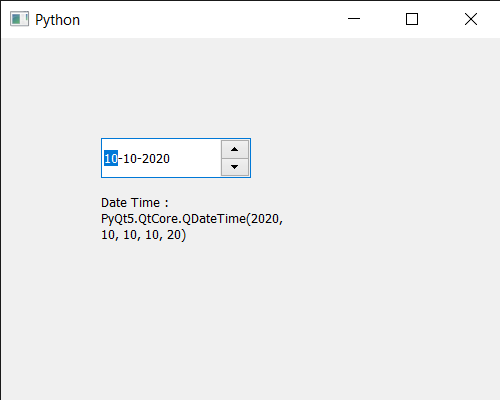Open In App

# PyQt5 QDateEdit – Getting Date Time

In this article we will see how we can get the date time of the QDateEdit. User can set date to the date edit with the help of cursor and the keyboard but sometimes there is a need of setting initial date or date programmatically for showing date in the records, which can be done with the help of setDate method. Unlike normal date, date time has date as well as the time although time is not shown on the date edit. Date time can set to the date edit with the help of setDate method. In order to do this we use dateTime method with the QDateEdit object

Syntax : date.dateTime()
Argument : It takes no argument
Return : It returns QDateTime object

Below is the implementation

## Python3

 `# importing libraries``from` `PyQt5.QtWidgets ``import` `*``from` `PyQt5 ``import` `QtCore, QtGui``from` `PyQt5.QtGui ``import` `*``from` `PyQt5.QtCore ``import` `*``import` `sys`  `class` `Window(QMainWindow):` `    ``def` `__init__(``self``):``        ``super``().__init__()` `        ``# setting title``        ``self``.setWindowTitle("Python ")` `        ``# setting geometry``        ``self``.setGeometry(``100``, ``100``, ``500``, ``400``)` `        ``# calling method``        ``self``.UiComponents()` `        ``# showing all the widgets``        ``self``.show()` `    ``# method for components``    ``def` `UiComponents(``self``):` `        ``# creating a QDateEdit widget``        ``date ``=` `QDateEdit(``self``)` `        ``# setting geometry of the date edit``        ``date.setGeometry(``100``, ``100``, ``150``, ``40``)` `        ``# date time``        ``d ``=` `QDateTime(``2020``, ``10``, ``10``, ``10``, ``20``)` `        ``# setting date time``        ``date.setDateTime(d)`  `        ``# creating a label``        ``label ``=` `QLabel("GeeksforGeeks", ``self``)` `        ``# setting geometry``        ``label.setGeometry(``100``, ``150``, ``200``, ``60``)` `        ``# making label multiline``        ``label.setWordWrap(``True``)` `        ``# getting date time``        ``value ``=` `date.dateTime()` `        ``# setting text to the label``        ``label.setText("Date Time : " ``+` `str``(value))`   `# create pyqt5 app``App ``=` `QApplication(sys.argv)` `# create the instance of our Window``window ``=` `Window()` `# start the app``sys.exit(App.``exec``())`

Output :